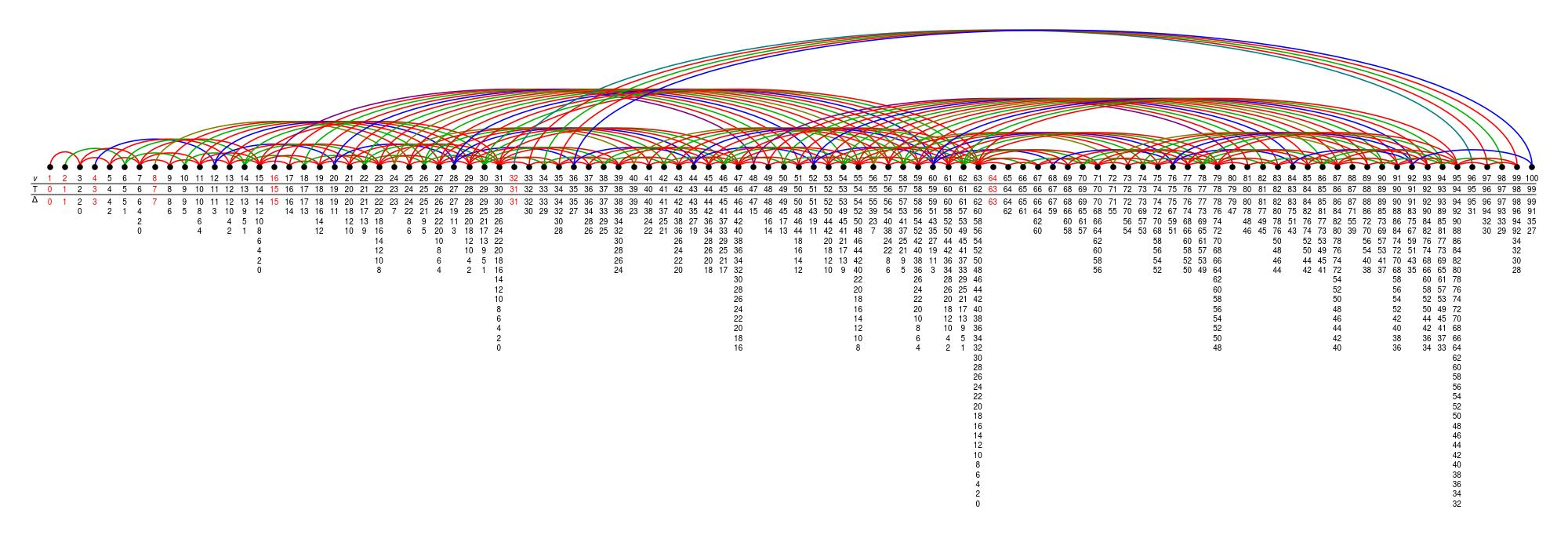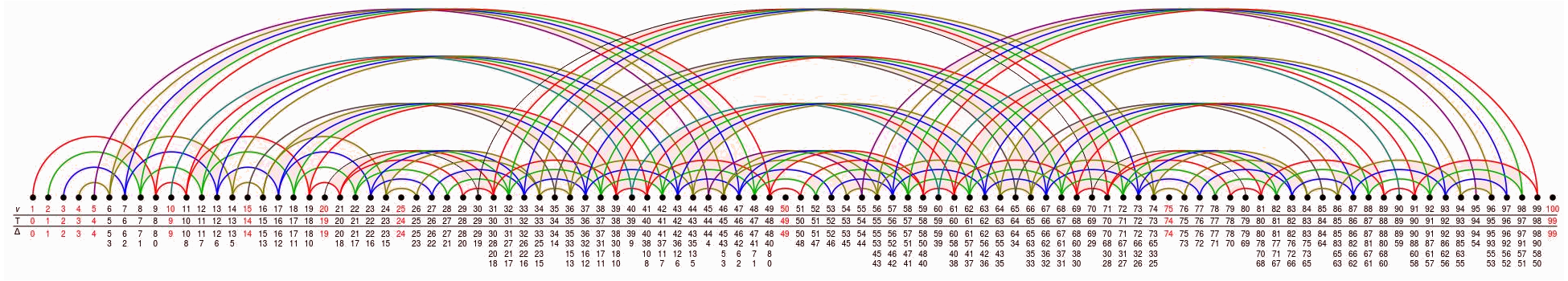## Data

• Title: Quivers for $$\mathrm{SL}_{2}$$ tilting modules
• Authors: Daniel Tubbenhauer and Paul Wedrich
• Status: Represent. Theory 25 (2021), 440-480. Last update: Mon, 31 May 2021 06:37:56 UTC
• ArXiv version = 0.99 published version
• LaTex Beamer presentation: Slides, Slides2, Slides3

## Abstract

Using diagrammatic methods, we define a quiver algebra depending on a prime $$\mathsf{p}$$ and show that it is the algebra underlying the category of tilting modules for $$\mathrm{SL}_{2}$$ in characteristic $$\mathsf{p}$$. Along the way, we obtain a presentation for morphisms between $$\mathsf{p}$$-Jones-Wenzl projectors.

## A few extra words

Let $$\mathbb{K}$$ denote an algebraically closed field and $$\mathbf{Tilt}=\mathbf{Tilt}\big(\mathrm{SL}_{2}(\mathbb{K})\big)$$ the additive, $$\mathbb{K}$$-linear category of (left-)tilting modules for the algebraic group $$\mathrm{SL}_{2}=\mathrm{SL}_{2}(\mathbb{K})$$. This category can be described as the full subcategory of $$\mathrm{SL}_{2}$$-modules which is monoidally generated by the vector representation $$T(1)\cong\mathbb{K}^{2}$$, and which is closed under taking finite direct sums and direct summands.
The purpose of this paper is to give a generators and relations presentation of $$\mathbf{Tilt}$$ by identifying it with the category of projective modules for an explicitly described quiver algebra. For $$\mathbb{K}$$ of characteristic zero this is trivial as $$\mathbf{Tilt}$$ is semisimple, and the indecomposable tilting modules are indeed the simple modules. The quantum analog at a complex root of unity is related to the zigzag algebra with vertex set $$\mathbb{N}$$ and a starting condition.
The focus of this paper is on the case of positive characteristic $$\mathsf{p}>0$$, for which we represent $$\mathbf{Tilt}$$ as a quotient $$Z=Z_{\mathsf{p}}$$ of the path algebra of an infinite, fractal-like quiver, a truncation of which is illustrated for $$\mathsf{p}=3$$ asThis illustrates the full subquiver containing the first 100 vertices of the quiver underlying $$Z_{3}$$.
Note that the algebra $$Z$$ contains information about the representation theory of $$\mathrm{SL}_{2}$$, as e.g. about the Weyl factors $$\Delta(w_{i}-1)$$ in $$T(v-1)$$. If the $$\mathsf{p}$$-adic expansion $$v=\sum_{i=0}^{j}a_{i}\mathsf{p}^{i}$$ has exactly r+1 non-zero digits, then there are $$2^{r}$$ such factors and, correspondingly, r arrows from v-1 to certain $$w_{i}-1 Note further the uniform behavior of \(Z$$ with respect to $$\mathsf{p}$$. For exampleis (a cut-off) of the quivers $$Z_{2}$$, $$Z_{5}$$ and $$Z_{7}$$, which, zooming out such that the precise labels get invisible, look basically the same as the one for $$Z_3$$.
The basis for our work is the classical fact that the Temperley-Lieb algebra controls the finite-dimensional representation theory of $$\mathrm{SL}_{2}$$. The second main ingredient is an explicit description of $$\mathsf{p}$$-Jones--Wenzl projectors, which are characteristic $$\mathsf{p}$$ analogs of the classical Jones-Wenzl projectors.
The bulk of this paper is devoted to a careful study of morphisms between $$\mathsf{p}$$-Jones--Wenzl projectors over $$\mathbb{F}_{\mathsf{p}}$$ and the linear relations between them; a result of which we think as being of independent interest.